• ## 系统函数,频率响应定义

万次阅读 多人点赞 2018-05-09 13:17:31
先求取冲激响应,在由公式(2)或(3)求取频率响应. 参考例1 此方法也就是对h(t)做傅里叶变换 第二种, 根据公式(1),令 x ( t ) = e j w t x ( t ) = e j w t x(t)=e^{jwt} , y ( t ) = H ( j w ) e j w t y ( t ) =...
基本概念

复指数集包括连续时间的est${e}^{st}$$e^{st}$ 和离散时间信号的zN${z}^{N}$$z^N$信号,其中s$s$$s$和z$z$$z$都是复数.一般来说,s$s$$s$和z$z$$z$可以是任意复数,但傅里叶分析仅限于这些变量的特殊形式.
在连续时间情况下仅涉及s$s$$s$的纯虚部值,即s=jw$s=jw$$s=jw$,因此仅考虑ejwt${e}^{jwt}$$e^{jwt}$形式的复指数.
在离散时间情况下仅限于单位振幅的z$z$$z$值,即z=ejw$z={e}^{jw}$$z=e^{jw}$,因此仅考虑ejwn${e}^{jwn}$$e^{jwn}$形式的复指数.
一个线性时不变系统的特性可以完全由它的冲激响应来决定
离散系统
y[n]=∑k=−∞+∞x[k]h[n−k]=x[k]∗h[k]$y\left[n\right]=\sum _{k=-\mathrm{\infty }}^{+\mathrm{\infty }}x\left[k\right]h\left[n-k\right]=x\left[k\right]\ast h\left[k\right]$y[n] = \sum_{k=-\infty}^{+\infty} x[k]h[n-k]=x[k]*h[k]
h[k]$h\left[k\right]$$h[k]$为y[k]$y\left[k\right]$$y[k]$的单位冲激响应
连续系统
y[t]=∫+∞−∞x[τ]h[t−τ]dτ=x[t]∗h[t]$y\left[t\right]={\int }_{-\mathrm{\infty }}^{+\mathrm{\infty }}x\left[\tau \right]h\left[t-\tau \right]d\tau =x\left[t\right]\ast h\left[t\right]$y[t] = \int_{-\infty}^{+\infty} x[τ]h[t-τ]dτ=x[t]*h[t]
h[t]$h\left[t\right]$$h[t]$为y[t]$y\left[t\right]$$y[t]$的单位冲激响应

线性时不变系统对复指数信号的响应

在研究线性时不变系统时,将信号表示为基本信号的线性组合是有利的,这些基本信号应该具有以下两个性质.

有这些基本信号能够构成相当广泛的一类有用信号
线性时不变系统对每一个基本信号的响应应该十分简单,以使系统对任意输入信号的响应有一个很方便的表达式
连续和离散时间信号集都具有上述两个性质,即连续时间的est${e}^{st}$$e^{st}$ 和离散时间信号的zN${z}^{N}$$z^N$信号,其中s$s$$s$和z$z$$z$都是复数.
在研究线性时不变系统时,复指数信号的重要性在于这样一个事实.即一个线性时不变系统对复指数信号的响应也是同样一个复指数信号,不同的只是幅度上的变换,即
est→H(s)estzN→H(z)zN(1)$\begin{array}{}\text{(1)}& {e}^{st}\to H\left(s\right){e}^{st}\phantom{\rule{0ex}{0ex}}{z}^{N}\to H\left(z\right){z}^{N}\end{array}$e^{st}\rightarrow H(s)e^{st} \\
z^N\rightarrow H(z)z^N \tag{1}
其中H(s)$H\left(s\right)$$H(s)$或H(z)$H\left(z\right)$$H(z)$是一个复振幅因子,一般来说是复变量s$s$$s$或z$z$$z$的函数.

对公式(1)的证明如下

连续时间系统,其单位冲激响应为h(t)$h\left(t\right)$$h(t)$,输入x(t)=est$x\left(t\right)={e}^{st}$$x(t)=e^{st}$
y(t)=∫+∞−∞h(τ)x(t−τ)dτ=∫+∞−∞h(τ)es(t−τ)dτ=est∫+∞−∞h(τ)e−sτdτ(1)(2)(3)$\begin{array}{}\text{(1)}& y\left(t\right)& ={\int }_{-\mathrm{\infty }}^{+\mathrm{\infty }}h\left(\tau \right)x\left(t-\tau \right)d\tau \text{(2)}& & ={\int }_{-\mathrm{\infty }}^{+\mathrm{\infty }}h\left(\tau \right){e}^{s\left(t-\tau \right)}d\tau \text{(3)}& & ={e}^{st}{\int }_{-\mathrm{\infty }}^{+\mathrm{\infty }}h\left(\tau \right){e}^{-s\tau }d\tau \end{array}$\begin{align}
y(t) &= \int_{-\infty}^{+\infty} h(τ)x(t-τ)dτ \\
&=\int_{-\infty}^{+\infty}h(τ)e^{s(t-τ)}dτ \\
&=e^{st} \int_{-\infty}^{+\infty}h(τ)e^{-sτ}dτ
\end{align}
令H(s)=∫+∞−∞h(τ)e−sτdτ$H\left(s\right)={\int }_{-\mathrm{\infty }}^{+\mathrm{\infty }}h\left(\tau \right){e}^{-s\tau }d\tau$$H(s)= \int_{-\infty}^{+\infty}h(τ)e^{-sτ}dτ$ ,假定H(s)$H\left(s\right)$$H(s)$收敛,于是系统对est${e}^{st}$$e^{st}$的响应为
y(t)=H(s)est$y\left(t\right)=H\left(s\right){e}^{st}$y(t)=H(s)e^{st}
其中H(s)$H\left(s\right)$$H(s)$是一个常复数,其值决定于s$s$$s$.
离散时间系统,其单位冲激响应为h(n)$h\left(n\right)$$h(n)$,输入x(n)=zn$x\left(n\right)={z}^{n}$$x(n)=z^n$
y(n)=∑k=−∞+∞h(k)x(n−k)=∑k=−∞+∞h(k)zn−k=∑k=−∞+∞znh(k)z−k(4)(5)(6)$\begin{array}{}\text{(4)}& y\left(n\right)& =\sum _{k=-\mathrm{\infty }}^{+\mathrm{\infty }}h\left(k\right)x\left(n-k\right)\text{(5)}& & =\sum _{k=-\mathrm{\infty }}^{+\mathrm{\infty }}h\left(k\right){z}^{n-k}\text{(6)}& & =\sum _{k=-\mathrm{\infty }}^{+\mathrm{\infty }}{z}^{n}h\left(k\right){z}^{-k}\end{array}$\begin{align}
y(n) &= \sum_{k=-\infty}^{+\infty} h(k)x(n-k) \\
&=\sum_{k=-\infty}^{+\infty} h(k)z^{n-k}  \\
&=\sum_{k=-\infty}^{+\infty} z^nh(k)z^{-k}
\end{align}
令H(z)=∑+∞k=−∞h(k)z−k$H\left(z\right)=\sum _{k=-\mathrm{\infty }}^{+\mathrm{\infty }}h\left(k\right){z}^{-k}$$H(z)= \sum_{k=-\infty}^{+\infty}h(k)z^{-k}$ ,假定H(z)$H\left(z\right)$$H(z)$收敛,于是系统对zn${z}^{n}$$z^n$的响应为
y(n)=H(z)zn$y\left(n\right)=H\left(z\right){z}^{n}$y(n)=H(z)z^n
其中H(z)$H\left(z\right)$$H(z)$是一个常数,其值决定于z$z$$z$.

系统函数/频率响应

当s和z是一般复数时,H(s)$H\left(s\right)$$H(s)$和H(z)$H\left(z\right)$$H(z)$就称为该系统的系统函数.
一般复数就是指:
在连续时间情况下仅涉及s的纯虚部值,即s=jw$s=jw$$s=jw$,因此仅考虑ejwt${e}^{jwt}$$e^{jwt}$形式的复指数.
在离散时间情况下仅限于单位振幅的zz值,即z=ejwz=ejw,因此仅考虑ejwnejwn形式的复指数.
对连续信号,具有s=jw$s=jw$$s=jw$形式的系统函数即H(s)=H(jw)$H\left(s\right)=H\left(jw\right)$$H(s)=H(jw)$就称为该系统的频率响应.
H(jw)=∫+∞−∞h(t)e−jwtdt(2)$\begin{array}{}\text{(2)}& H\left(jw\right)={\int }_{-\mathrm{\infty }}^{+\mathrm{\infty }}h\left(t\right){e}^{-jwt}dt\end{array}$H(jw)= \int_{-\infty}^{+\infty}h(t)e^{-jwt}dt\tag{2}
对离散信号,具有z=ejw$z={e}^{jw}$$z=e^{jw}$形式的系统函数即H(z)=H(ejw)$H\left(z\right)=H\left({e}^{jw}\right)$$H(z)=H(e^{jw})$就称为该系统的频率响应.
H(ejw)=∑k=−∞+∞h(k)e−jwk(3)$\begin{array}{}\text{(3)}& H\left({e}^{jw}\right)=\sum _{k=-\mathrm{\infty }}^{+\mathrm{\infty }}h\left(k\right){e}^{-jwk}\end{array}$H(e^{jw})=  \sum_{k=-\infty}^{+\infty}h(k)e^{-jwk}\tag{3}

求频响方法

第一种,先求取冲激响应,在由公式(2)或(3)求取频率响应. 参考例1
此方法也就是对h(t)做傅里叶变换
第二种,根据公式(1),令x(t)=ejwt$x\left(t\right)={e}^{jwt}$$x(t)=e^{jwt}$,y(t)=H(jw)ejwt$y\left(t\right)=H\left(jw\right){e}^{jwt}$$y(t)=H(jw)e^{jwt}$,将其代入微分方程求解. 参考例2

例1.

考虑一个线性时不变系统,它在输入值上连续取2点平均,求其频率响应
y[n]=12(x[n]+x[n−1])$y\left[n\right]=\frac{1}{2}\left(x\left[n\right]+x\left[n-1\right]\right)$y[n] = \frac{1}{2}(x[n]+x[n-1])
解:冲激响应为(代入x[n]=δ[n]$x\left[n\right]=\delta \left[n\right]$$x[n]=\delta[n]$)
h[n]=12(δ[n]+δ[n−1])$h\left[n\right]=\frac{1}{2}\left(\delta \left[n\right]+\delta \left[n-1\right]\right)$h[n]=\frac{1}{2}(\delta[n]+\delta[n-1])
由公式(3),其频率响应为
H(ejw)=∑k=−∞+∞h(k)z−jwk=∑k=−∞+∞12(δ[k]+δ[k−1])z−jwk=12(1+e−jw)=12(1+cosw−jsinw)=12(2cos2w2−2jsinw2cosw2)=cosw2(cosw2−sinw2)=e−jw2cosw2(77)(78)(79)(80)(81)(82)(83)$\begin{array}{}\text{(77)}& H\left({e}^{jw}\right)& =\sum _{k=-\mathrm{\infty }}^{+\mathrm{\infty }}h\left(k\right){z}^{-jwk}\text{(78)}& & =\sum _{k=-\mathrm{\infty }}^{+\mathrm{\infty }}\frac{1}{2}\left(\delta \left[k\right]+\delta \left[k-1\right]\right){z}^{-jwk}\text{(79)}& & =\frac{1}{2}\left(1+{e}^{-jw}\right)\text{(80)}& & =\frac{1}{2}\left(1+cosw-jsinw\right)\text{(81)}& & =\frac{1}{2}\left(2co{s}^{2}\frac{w}{2}-2jsin\frac{w}{2}cos\frac{w}{2}\right)\text{(82)}& & =cos\frac{w}{2}\left(cos\frac{w}{2}-sin\frac{w}{2}\right)\text{(83)}& & ={e}^{-\frac{jw}{2}}cos\frac{w}{2}\end{array}$\begin{align}
H(e^{jw})&=  \sum_{k=-\infty}^{+\infty}h(k)z^{-jwk} \\
&=  \sum_{k=-\infty}^{+\infty}\frac{1}{2}(\delta[k]+\delta[k-1])z^{-jwk} \\
&=\frac{1}{2}(1+e^{-jw}) \\
&= \frac{1}{2}(1+cosw - jsinw) \\
&= \frac{1}{2}(2cos^2\frac{w}{2} - 2jsin\frac{w}{2}cos\frac{w}{2}) \\
&= cos\frac{w}{2}(cos\frac{w}{2}-sin\frac{w}{2}) \\
&= e^{-\frac{jw}{2}}cos\frac{w}{2} \\
\end{align}
H(ejw)$H\left({e}^{jw}\right)$$H(e^{jw})$幅相曲线如下图所示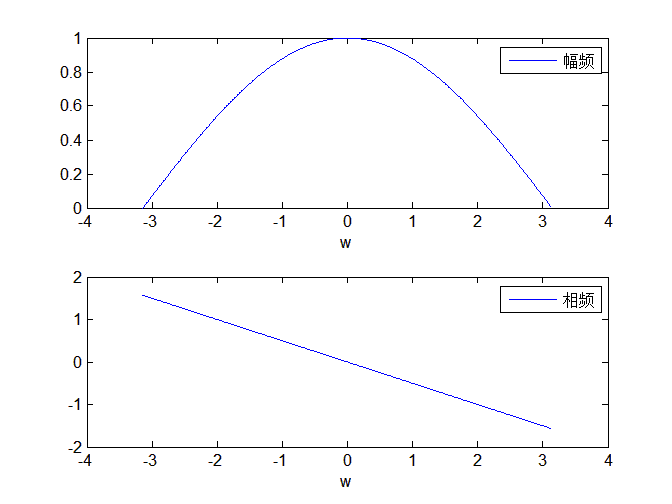matlab代码如下

clear all;close all; clc;
H = @(w) exp(-1j*w/2).*cos(w/2);
w = (-pi:0.01:pi);
val = H(w);
subplot(211)
plot(w, abs(val));
xlabel('w'); legend('幅频');
subplot(212)
plot(w, angle(val));
xlabel('w'); legend( '相频'); 

例2.

一阶连续系统,其微分方程如下,求其频率响应
τdy(t)dt+y(t)=x(t)$\tau \frac{dy\left(t\right)}{dt}+y\left(t\right)=x\left(t\right)$τ\frac{dy(t)}{dt}+y(t)=x(t)
解.令x(t)=ejwt$x\left(t\right)={e}^{jwt}$$x(t)=e^{jwt}$,y(t)=H(jw)ejwt$y\left(t\right)=H\left(jw\right){e}^{jwt}$$y(t)=H(jw)e^{jwt}$,将其代入微分方程
τd[H(jw)ejwt]dt+H(jw)ejwt=ejwt⇒jwτH(jw)ejwt+H(jw)ejwt=ejwt⇒H(jw)=1jwτ+1$\tau \frac{d\left[H\left(jw\right){e}^{jwt}\right]}{dt}+H\left(jw\right){e}^{jwt}={e}^{jwt}\phantom{\rule{1em}{0ex}}⇒\phantom{\rule{0ex}{0ex}}jw\tau H\left(jw\right){e}^{jwt}+H\left(jw\right){e}^{jwt}={e}^{jwt}\phantom{\rule{1em}{0ex}}⇒\phantom{\rule{0ex}{0ex}}H\left(jw\right)=\frac{1}{jw\tau +1}$τ\frac{d[H(jw)e^{jwt}]}{dt}+H(jw)e^{jwt}=e^{jwt}\quad \Rightarrow \\
H(jw) = \frac{1}{jwτ+1}


展开全文• 从浏览器 和 服务器的角度，可以给出响应时间的不同定义。 浏览器角度的HTTP API响应时间SLA定义 站在浏览器的角度，从开始发送请求数据的时刻开始计算响应时间，到浏览器响应数据接收完毕的时刻为止。 其
HTTP (RESTful) API 响应时间分析及SLA定义
author: archimekai
HTTP API时间过程分解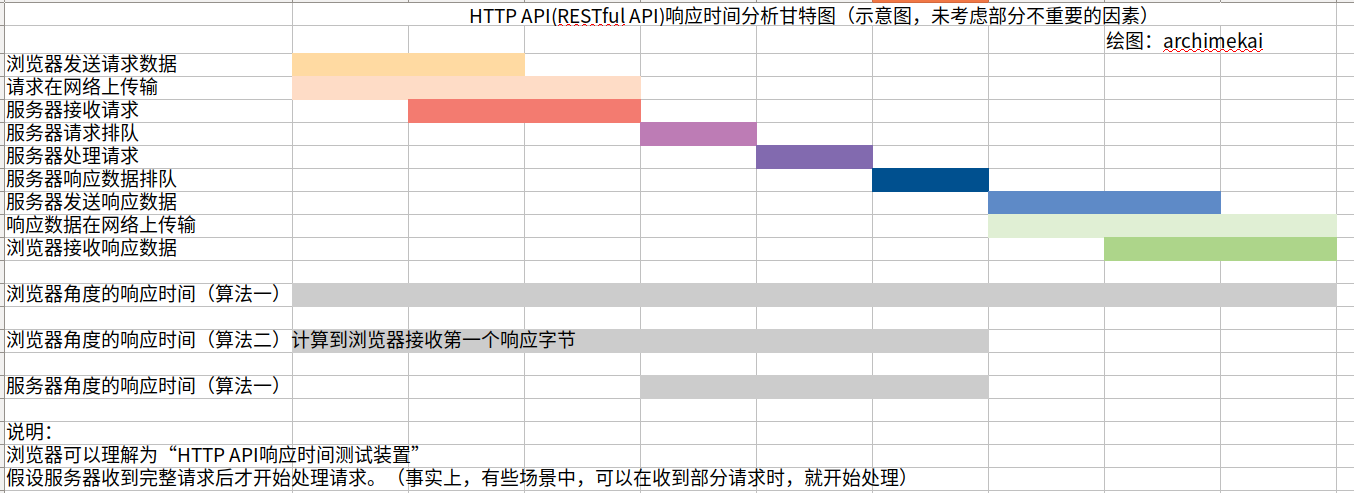TODO 各时间阶段分析
浏览器接受请求数据
如果浏览器边接收请求数据边处理，浏览器对请求数据的处理速度可能会影响浏览器接收请求数据的速度。
HTTP API响应时间定义
从浏览器 和 服务器的角度，可以给出响应时间的不同定义。
浏览器角度的HTTP API响应时间SLA定义
站在浏览器的角度，从开始发送请求数据的时刻开始计算响应时间，到浏览器响应数据接收完毕的时刻为止。
其包括的时间如下：
浏览器发送请求数据
请求在网络上传输
服务器接收请求
服务器请求排队
服务器处理请求
服务器响应数据排队
服务器发送响应数据
响应数据在网络上传输
浏览器接收响应数据
服务器角度的HTTP API响应时间SLA定义
站在服务器的角度，从请求接收完毕的那一刻开始计算响应时间，到服务器开始发送响应数据的那一刻为止。
其包括的时间如下：
服务器请求排队
服务器处理请求
服务器响应数据排队
TODO 用户角度的响应时间定义


展开全文• 两个基本信号 δ(t)，ϵ(t)\delta (t)，\epsilon(t)δ(t)，ϵ(t) 冲激信号的定义和求法 冲激响应是由单位冲激函数 δ(t)\delta(t)δ(t)所引起的零状态响应，记为 h(t)h(t)h(t)。 h(t)h(t)h(t)隐含的条件： f(t)=δ(t...
两个基本信号$\delta (t)，\epsilon(t)$
冲激信号的定义和求法
冲激响应是由单位冲激函数$\delta(t)$所引起的零状态响应，记为$h(t)$。$h(t)$隐含的条件：
$f(t) = \delta(t)$
$h(0_-)=h'(0_-)=0{(对二阶系统)}$
基本信号：冲激函数$\delta(t)$
基本响应：冲激响应$h(t)$
求法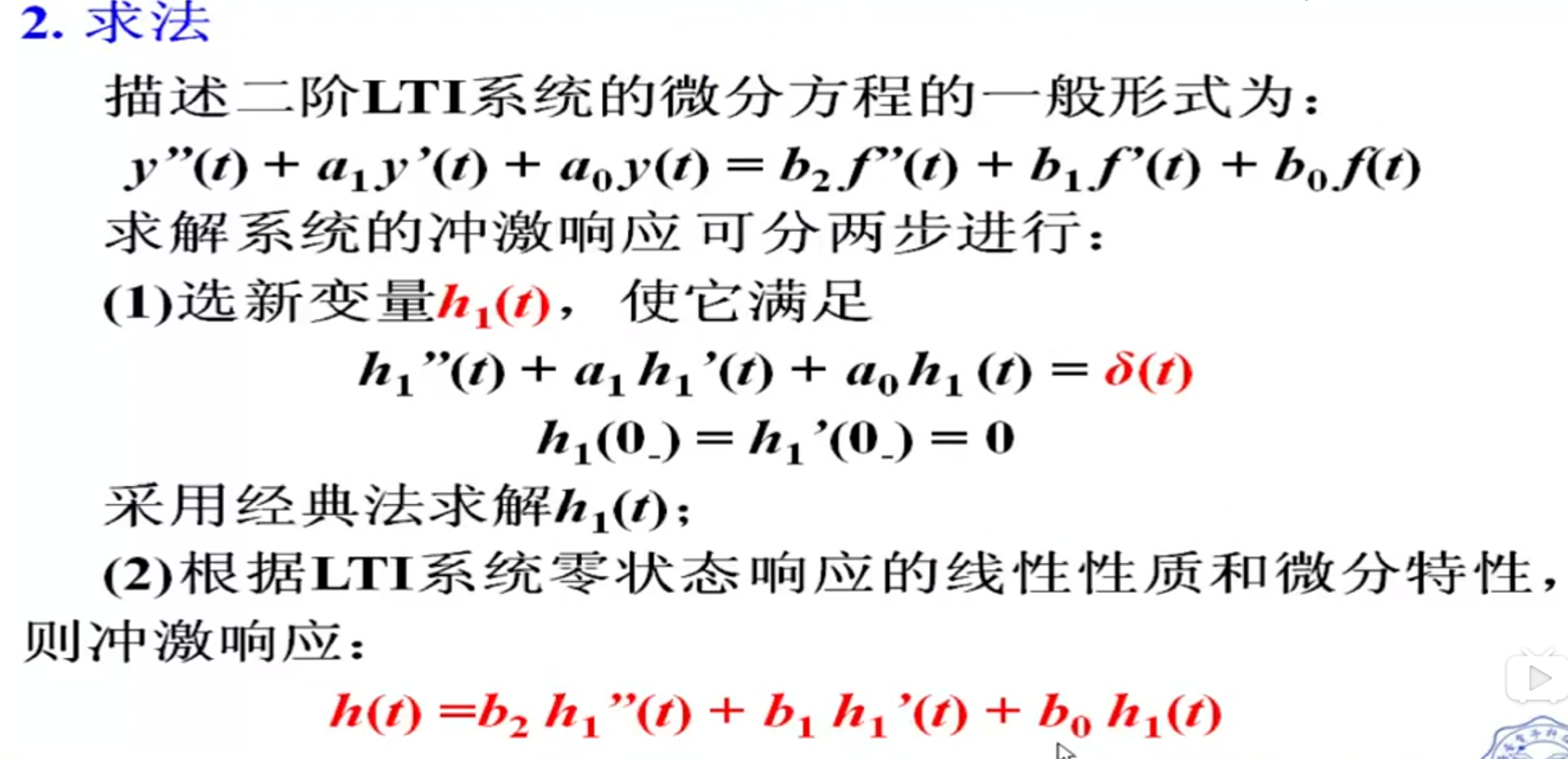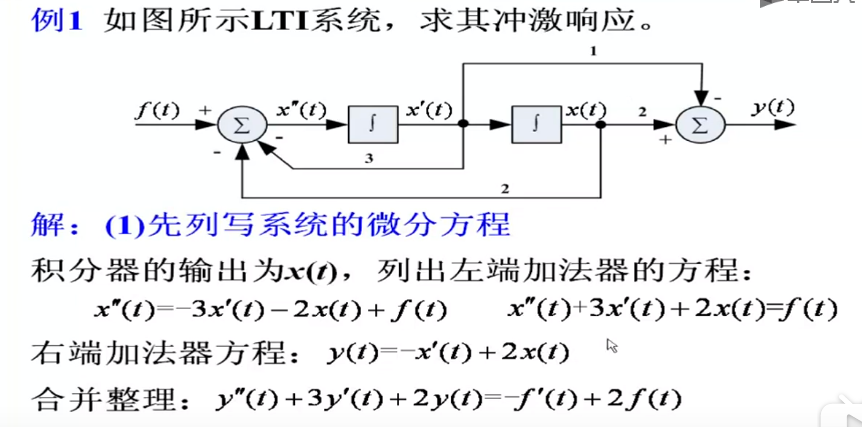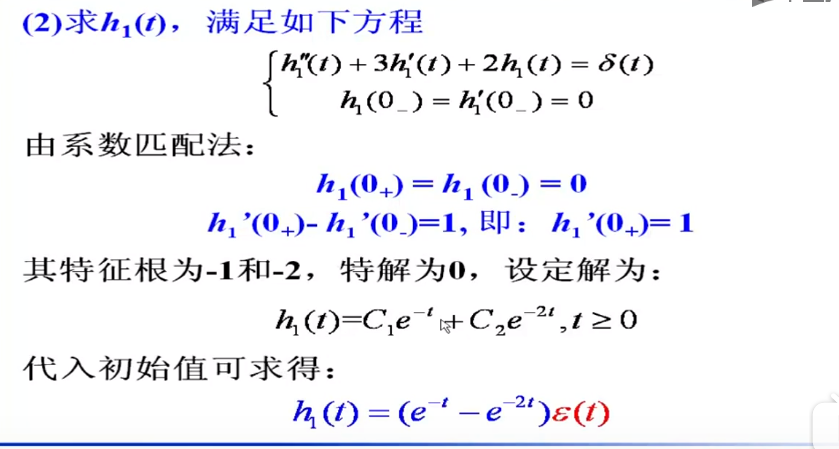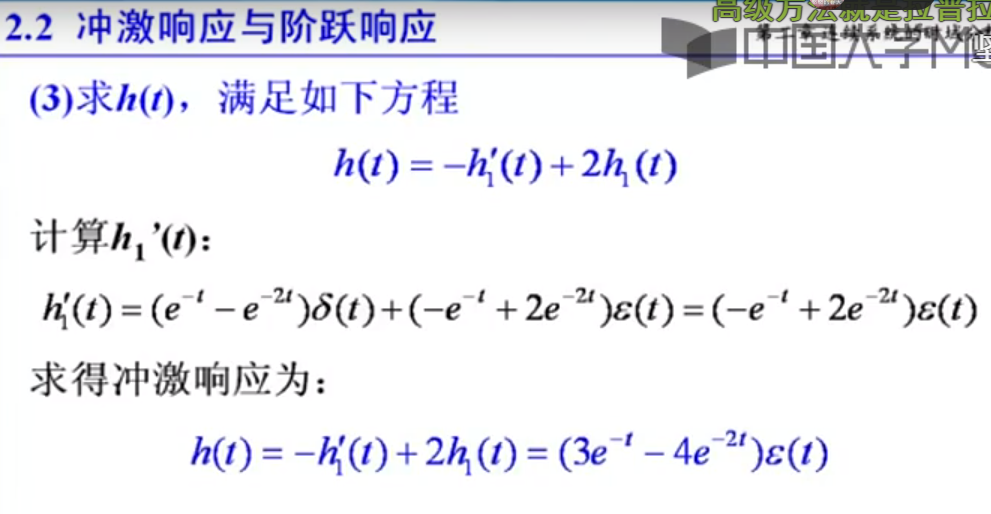说明：结合零状态响应的线性性质和微分性质，来简化求解过程；如直接进行求解，方程右端将会出现冲激函数的各阶导数。


展开全文• // 定义按钮的响应函数 $('#aButton').on('click', function(){ alert('test'); }); // 定义按钮的响应函数$('#aButton').on('click', function(){
});

展开全文Python flask web框架 json
• 俗话说无规矩不成方圆，一个接口一种数据响应格式，一个开发人员一种开发格式，导致代码不规范，数据解析复杂化，维护更是难上加难。 1.关于接口响应数据格式参考[json格式],做到不使用的属性不返回 基础响应格式...经验分享 json 开发人员
• ## 单位阶跃响应求解

千次阅读 2020-11-17 09:26:25
单位阶跃响应定义：系统在单位阶跃信号的作用下所产生的零状态响应。 例： 求单位阶跃响应。 方法一：求出系统的传递函数，设单位阶跃输入的拉氏变换为，则单位阶跃响应的拉氏变换为: 则单位阶跃响应为： ...
• 原因： 使用jquery定义按钮触发click事件，不能够响应，是因为页面在装载时候，是一行一行解析的并且只解析一次，所以在页面装载后操作你需要执行的按钮，无法触发事件 解决方法：把需要触发事件的方法写在$("docujquery function button input • 在Qt开发里面，有一种传说中的信号槽机制，有好几种实现的方法。...可以在模式下面，自己定义信号和槽函数，然后在窗口类里面，把相应的槽函数定义好 2 可以纯代码实现。把ui的控件拖动好，把槽函数写好，然后用一句c • 但示例中的响应报文不符合我们定义的数据规范，想要使其满足自己的数据规范，可以自己定义一个新的 ErrorController，代码如下： @Controller @RequestMapping ( "${server.error.path:\${error.path:/error}}" ...
• MFC也定义了丰富的宏来简化消息响应的代码。这使得很多初学者都能快速开发出基于消息响应机制的应用程序。然后也正是这些带来方便的宏，使很多人摸不着头脑。当不小心的代码操作使自动化工具不好用的时候，看着那一...command struct pascal
• 出现此问题的原因是：你刚打开VS时提示的错误没有解决。 错误图片如下： 不必惊慌，不用重装，找到VS的安装包（光盘映像文件），打开后搜索SSCERuntime_x86-chs.msi 并安装； 如果还不行，可以试试安装这个 ...控件
• 零输入响应与零状态响应零输入响应与零状态响应1、 零输入响应2、零状态响应3、两种响应的区别4、两种响应的判断方法5、两种响应的求解方法6、两种响应之间的联系 零输入响应与零状态响应 这两个响应在大学的电路中...信号与系统
• 定义 结构 请求方式 GET和POST区别 响应 定义 结构 响应状态码 ​ 请求 Request 浏览器根据用户请求方式，将请求相关数据按照HTTP规定的格式进行封装，然后将封装好的数据发送给指定服务器。 定义 ...java javaweb http
• 前两天，vs2010“转到定义”功能突然没用了，屏幕闪一下就没了，而且f12也没用。重装修复了两次vs2010也没有解决。   网上搜了一些资料，大致讲的解决方法有两种，一种是全部重置vs2010的设置，另一种就是重新...
• ## HTTP 响应代码全集

千次阅读 多人点赞 2019-10-15 15:01:20
HTTP 响应状态代码指示特定 http请求是否已成功完成。...状态代码由section 10 of RFC 2616定义 信息响应 100 Continue 这个临时响应表明，迄今为止的所有内容都是可行的，客户端应该继续请...
• ## 响应式布局

千次阅读 2015-03-20 10:01:37
今天就和大家来讲讲响应式布局这件小事，包含什么是响应式布局、响应式布局的优点和缺点以及响应式布局该怎么设计（通过CSS3 Media Query实现响应布局）。 看页面点击这里一、什么是响应式布局? 响应式布局是Et分辨率 布局
• （为何不全为0，试想，如果初值全为0，输入也为0，那就不用求输出信号了，因为输出信号肯定也是0） 零状态响应定义：已知状态变量的初值在t=0-时刻均为0，求给定输入信号x(t)作用下，系统的响应，也即：先求非齐次...
• 本系列其他文章见：《响应式Spring的道法术器》。 前情提要：响应式流 | lambda与函数式 | Reactor快速上手 1.3.3 Spring WebFlux Spring WebFlux是随Spring 5推出的响应式Web框架。 1）服务端技术栈 ......